# Part 2: Titanic — model building, scikit-learn

I started the Titanic project with the data analysis part, you can read the text here and see both notebooks in Github.

The next step was to build the model which predicts if the person survived or not. I did several models just to test out which model type is the best. Also, it was a great way to learn scikit-learn and Fast.ai packages

Model building was much harder than the initial data analysis part but during the model-building part, I learned several new things.

I created separate notebook for model building part so that’s why I started with basics again.

`# Upload the packagesimport pandas as pdimport numpy as np`

After loading necessary packages I loaded the data:

`df=pd.read_csv("train.csv")`

Then I had a quick look at the data:

`df.head()#This prints you the first 5 rows of the table`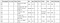Screenshot of the first rows
`df.tail()# This prints you out the last five rows of the tabledf.shape`

# Replace missing values

First of all, I started to prepare the data set which I used for model training. It means that I chose which features to use and which not.

I started to check how many missing values are there.

`df.isnull().sum()# Count missing values in each column`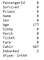Most of the Cabin values are missing

Then I thought that what to do with all missing values so that they wouldn’t affect the model accuracy in a negative way. I chose to fill missing Age values with the mean value.

`df['Age'] = df['Age'].fillna((df['Age'].mean()))# Fills missing values in "Age" -column with the averages values.`

There were only a few missing embarked value so I chose to fill it with last previous row value.

`df["Embarked"].fillna( method ='ffill', inplace = True)# Missing values are filled with previous row value`

Most of the cabin columns are missing values so I chose to add just value “empty” there.

`df["Cabin"].fillna("Empty", inplace = True)# All missing values in column "Cabin" are filled with word "empty"`

# Preprocessing data

Preprocessing the data is important because it helps to build models that are as accurate as possible.

`from sklearn import preprocessing# this package provides common utility functions and transformer classes to change raw feature vectors into a representation that is more suitable for the downstream estimators.# This package can be uploaded in the beginning together with pandas and numpy. `

I processed the data with get_dummies.

`df = pd.get_dummies(df, prefix_sep='_', drop_first=True)df.head()# prefix_sep='_' - If appending prefix, separator/delimiter to use.# drop_first=True - Whether to get k-1 dummies out of k categorical levels by removing the first level.`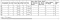Get_dummies convert categorical variables into dummy/indicator variables.

Using get_dummies makes values easier to quantify and compare. Dummy variables are needed for model training to separate different values of categorical variables.

# Training and test sets

The next step is to create separate datasets for training and testing.

`from sklearn.model_selection import train_test_split# Split arrays or matrices into random train and test sets# This can be added also to first row where pandas and numpy are uploaded.`

First of all, I need to choose what is the dependent variable I want to train a model for. As my goal is to predict whether a person survived I choose this value to be the “Survived” -column.

`y =df["Survived"]`

Then I choose which columns are used for the training set.

`df_train_input=df.loc[:, df.columns != 'Survived']# Training set is built by using all the columns but excluding column "Survived". `

After choosing the columns it’ s time to create training and testing sets.

`X_train, X_test, y_train, y_test = train_test_split(df_train_input, y, test_size=0.2)print (X_train.shape, y_train.shape)print (X_test.shape, y_test.shape)`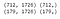Printed results

I created the following sets: X_train, X_test, y_train, and y_test. For the train set, I took columns that I defined earlier and for the test set I took 20% of data in the training set.

# Building the model

Finally, it is time to start to train the model. I used Scikit-learn for the model building but there are also several other ways to build the model.

I started with Gaussian Naive Bayes. It is a simple classification algorithm and based on the Bayes’ theorem.

`from sklearn.naive_bayes import GaussianNBfrom sklearn.metrics import accuracy_scoregnb = GaussianNB()pred=gnb.fit(X_train, y_train).predict(X_test)print("Naive-Bayes accuracy : ",accuracy_score(y_test, pred, normalize = True))`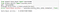Accuracy is very low, only 46%

Also, I tested LinearSVC which means Linear Support Vector Classification.

`from sklearn.svm import LinearSVCfrom sklearn.metrics import accuracy_scoresvc_model = LinearSVC(random_state=0)pred = svc_model.fit(X_train, y_train).predict(X_test)print("LinearSVC accuracy : ",accuracy_score(y_test, pred, normalize = True))`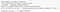Accuracy with LinearSVC model was much better, 73%

And last model type I tried was the K-Neighbors classifier.

`from sklearn.neighbors import KNeighborsClassifierfrom sklearn.metrics import accuracy_scoreneigh = KNeighborsClassifier(n_neighbors=3)neigh.fit(X_train, y_train)pred = neigh.predict(X_test)print ("KNeighbors accuracy score : ",accuracy_score(y_test, pred))`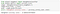Neither this accurate was good, only 58%.

As you can see none of these models was very good. I was not satisfied with the results so I started to learn how to extract features for building a better model. Here I used all the features just as they were in CSV-file but I realized it’s no good way to build the model.

Thank you for the highly trained monkey (Risto Hinno) for editing my texts and inspiring me!